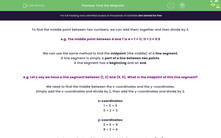# Find the Midpoint

In this worksheet, students will find the coordinates of midpoints of line segments with coordinates involving both numbers and algebra, or use knowledge of midpoints to find missing end or starting points.Key stage:  KS 4

Year:  GCSE

GCSE Subjects:   Maths

GCSE Boards:   Pearson Edexcel, OCR, Eduqas, AQA,

Curriculum topic:   Algebra, Graphs of Equations and Functions

Curriculum subtopic:   Graphs Straight Line Graphs

Difficulty level:#### Worksheet Overview

To find the middle point between two numbers, we can add them together and then divide by 2.

e.g. The middle point between 4 and 7 is 4 + 7 = 11, 11 ÷ 2 = 5.5

We can use the same method to find the midpoint (the middle) of a line segment.

A line segment is simply a part of a line between two points.

A line segment has a beginning and an end.

e.g. Let's say we have a line segment between (1, 2) and (5, 6). What is the midpoint of this line segment?

We need to find the middle between the x-coordinates and the y-coordinates.

Simply add the x-coordinates and divide by 2, then add the y-coordinates and divide by 2.

x-coordinates:

1 + 5 = 6

6 ÷ 2 = 3

y-coordinates:

2 + 6 = 8

8 ÷ 2 = 4

So the coordinates of the midpoint of this line segment are (3, 4).

In this activity, we will find the midpoints of line segments with coordinates involving both numbers and algebra, or use our knowledge of midpoints to find missing end or starting points.

### What is EdPlace?

We're your National Curriculum aligned online education content provider helping each child succeed in English, maths and science from year 1 to GCSE. With an EdPlace account you’ll be able to track and measure progress, helping each child achieve their best. We build confidence and attainment by personalising each child’s learning at a level that suits them.

Get started